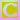Go to content
The vector AB is multiplied by a scalar to produce the vector AC. Also displayed are a = AB, b = 2AB, c = 3AB and d = -2AB. Please enable Java for an interactive construction (with Cinderella).
Please enable Java for an interactive construction (with Cinderella).Uses Cinderella interactive geometry

## Glossary

### scalar

A mathematical object with one property of magnitude or size.

### vector

A mathematical object with magnitude and direction.

Full Glossary List

## This question appears in the following syllabi:

SyllabusModuleSectionTopicExam Year
AQA A-Level (UK - Pre-2017)C4Vectors2D Vector geometry-
CBSE XII (India)Vectors and 3-D GeometryVectorsVectors and scalars, magnitude and direction of a vector-
CCEA A-Level (NI)C4Vectors2D Vector geometry-
CIE A-Level (UK)P1Vectors2D Vector geometry-
Edexcel A-Level (UK - Pre-2017)C4Vectors2D Vector geometry-
I.B. Higher Level4Vectors2D Vector geometry-
I.B. Standard Level4Vectors2D Vector geometry-
Methods (UK)M4Vectors2D Vector geometry-
OCR A-Level (UK - Pre-2017)C4Vectors2D Vector geometry-
OCR-MEI A-Level (UK - Pre-2017)C4Vectors2D Vector geometry-
Pre-Calculus (US)E1Vectors2D Vector geometry-
Pre-U A-Level (UK)6Vectors2D Vector geometry-
Scottish (Highers + Advanced)HM3Vectors2D Vector geometry-
Scottish HighersM3Vectors2D Vector geometry-
Universal (all site questions)VVectors2D Vector geometry-
WJEC A-Level (Wales)C4Vectors2D Vector geometry-